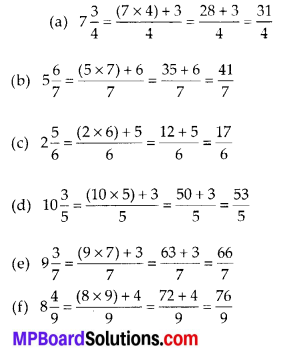## MP Board Class 6th Maths Solutions Chapter 7 Fractions Ex 7.2

Question 1.
Draw number lines and locate the points on them: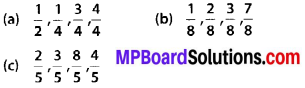Solution: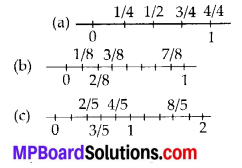Question 2.
Express the following as mixed fractions: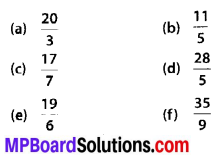Solution: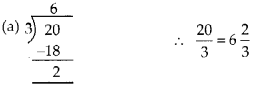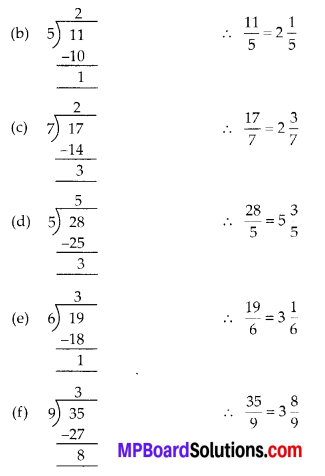Question 3.
Express the following as improper fractions: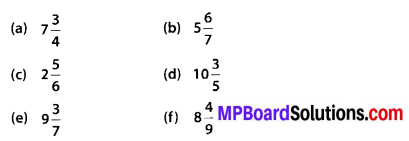Solution: